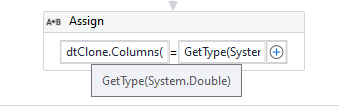# Convert column dt string to double

Good day everyone!
Please tell me how to convert the “Sum” column from string to double (empty strings may occur)?
Using For each row will take a very long time since there are about 20,000 rows in the database. Thanks.Tried to clone the dt and change the format but it didn’t work as there might be empty rows in the “Sum” column in the database.
dtClone.xaml (8.0 KB)

use this linq query

sum_data= dt1.AsEnumerable().Sum(Function(r) If(r(“Sum”).ToString.Trim.Equals(“”),0,CDbl(r(“Sum”).ToString)))

sum_data should be of type system.double

adding a updated file of yours here
dtClone.xaml (7.4 KB)

Regards,
Nived N

Thanks for the help, but it converts the LINQ query incorrectly.
The decimal places must be preserved.

@sereganator
Conversion can be done as described here:

Just your locals if , is decimal seperator

• add a new Column to the datatable ColumnName: dSum DataType: Double
• do the conversion e.g. with For Each Row, LINQ, Inoke Code incl. Handling Null, empty, invalid values
• remove old sum datacolumn
• move dSum col to other position
• rename dSum Col name to Sum

@sereganator If you are taking the values from database just convert the column in the SQL query itself.

Can I have an example please? More precisely, I do not know how to handle empty lines.

Hi @sereganator ,

Here is an activity to convert the column data type.

Regards
Balamurugan.S

Thanks, but need to convert without third party activities.

@sereganator

Here is the sql query

`Select Name, Age, Case When Sum <> '' And Sum is not null Then Cast(Sum as decimal(18,2)) Else 0 End As Sum From TableName`

Can still do as in my example, only on the right side write processing of zero values. It can be done?dtClone.xaml (8.0 KB)